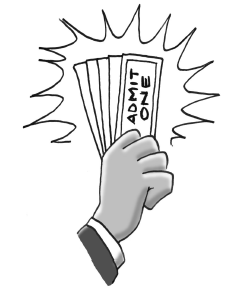### Home > AC > Chapter 11 > Lesson 11.1.1 > Problem11-8

11-8.When a family with two adults and three children bought tickets for an amusement park, they paid a total of $56.50$. The next family in line, with four children and one adult, paid $49.50$. Find the adult and child ticket prices by writing and solving a system of equations.

Write an equation for each family, where $x=\text{the price per adult}$ and $y=\text{the price per child}$.

The equation for the first family is: $2x + 3y = 56.50$
Write the equation for the other family and solve the system.